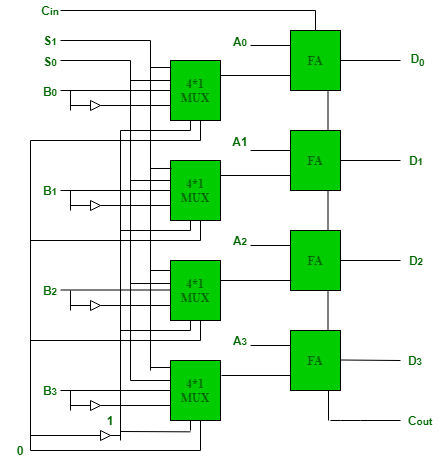# Arithmetic Circuits

• Difficulty Level : Medium
• Last Updated : 15 Feb, 2021

Arithmetic circuits can perform seven different arithmetic operations using a single composite circuit.
It uses a full adder (FA) to perform these operations. A multiplexer (MUX) is used to provide different inputs to the circuit in order to obtain different arithmetic operations as outputs.

4-bit Arithmetic Circuit :
Consider the following 4-bit Arithmetic circuit with inputs A and B. It can perform seven different arithmetic operations by varying the inputs of the multiplexer and the carry (C0).Truth Table for the above Arithmetic Circuit :

0

0

0

B

A + B

0

0

1

B

A + B + 1

0

1

0

B’

A + B’

0

1

1

B’

A + B’ + 1 = A – B

1

0

0

0

A

1

0

1

0

A + 1

1

1

0

1

A – 1

1

1

1

1

A – 1 + 1 = A

Hence, the different operations for the inputs A and B are –